# How to fix common JavaScript errors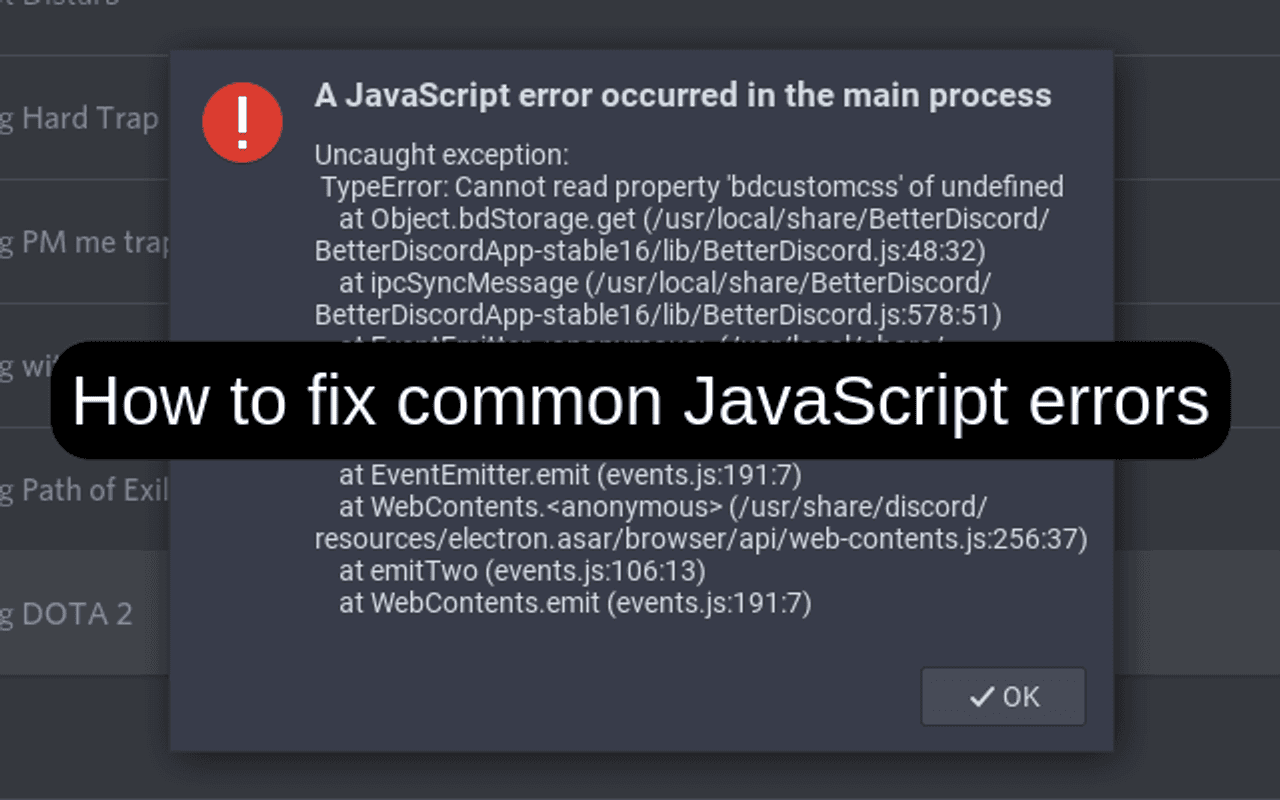Errors in JavaScript are quite difficult to track and fix. Each error has its own name. This helps with identifying the problem and fixing it. Untreated errors are displayed in the web console of the browser or the output of the Node.js application. Let's look at the types of common errors in JavaScript, why they occur, and how to fix them. Examples are shown for each type to improve your understanding of the error and actions to fix it.

There is no unified standard for error naming in different browsers. For this article we took error names from the Google Chrome browser.

## TypeError: Cannot read property “x” of “y”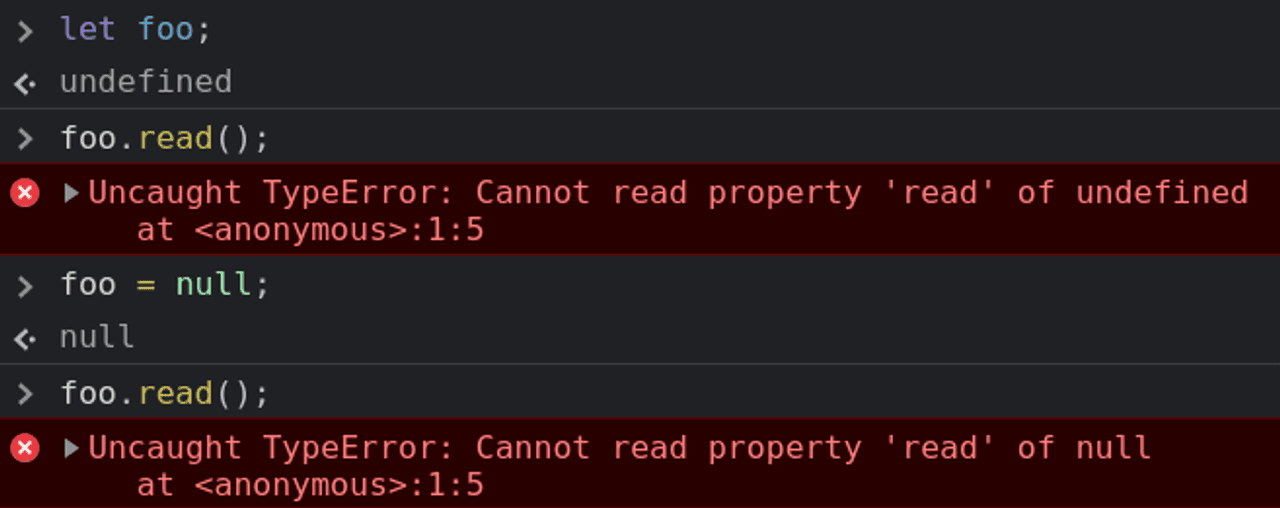This error occurs when we call a method or read a property on `undefined` or `null` values.

Safari has a different name for these errors:

• ‘undefined’ is not an object
• ‘null’ is not an object

In the Mozilla Firefox browser:

• TypeError: "x" is undefined
• TypeError: "x" is null

### Examples of errors

• Function call on `undefined`:
``let foo;foo.read(); // TypeError: Cannot read property 'read' of undefined``
• Read property on `null`:
``foo = null;foo.total; // TypeError: Cannot read property 'total of null``

### Error fix

There are several fixes, depending on the context of the issue.

• Set the value of a variable or a property of an object before using it.
``let foo = {  total: 5};let total = foo.total; // 5``
• Check that the variable is defined before using it
``if (typeof foo !== 'undefined' && typeof foo.total !== 'undefined') {  let total = foo.total;}``

## TypeError: “x” is not a functionAn error occurs when we try to call an undefined function.

### Examples of errors

There are several options for fixing it, depending on the causes of the error:

• The function is undefined.
``let x = {};x.read(); // TypeError: x.read is not a function``

In this example, let's define the `read()` function.

``let x = {  read: function() {    return 2;  }};x.read(); // 2``
• There is a typo in the function name in the place where the function is called or declared.
``let a = {  sam: function(a, b) {    return a + b;  }}a.sum(1, 1); // TypeError: a.sum is not a function``

After correcting a typo in the function name `sum()`.

``let a = {  sum: function(a, b) {    return a + b;  }}a.sum(1, 1); // 2``

## TypeError: Cannot set property “x” of undefined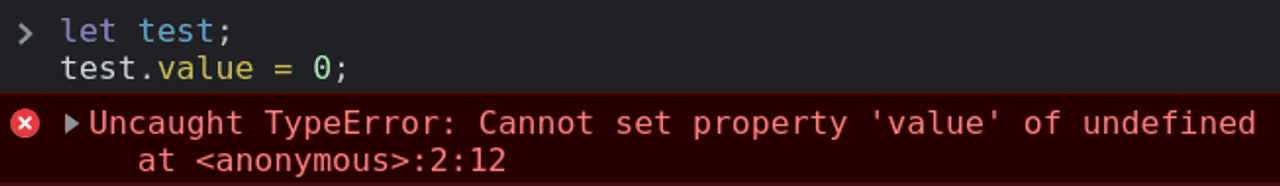This error occurs when we try to write values into the `undefined` property.

``let test;test.value = 0; // Uncaught TypeError: Cannot set property 'value' of undefined``

The solution in this example would be to initialize the variable `test` with a new object like this:

``let test = {};test.value = 0;``

Or like this:

``let test = {  value: 0};``

## ReferenceError: “x” is not defined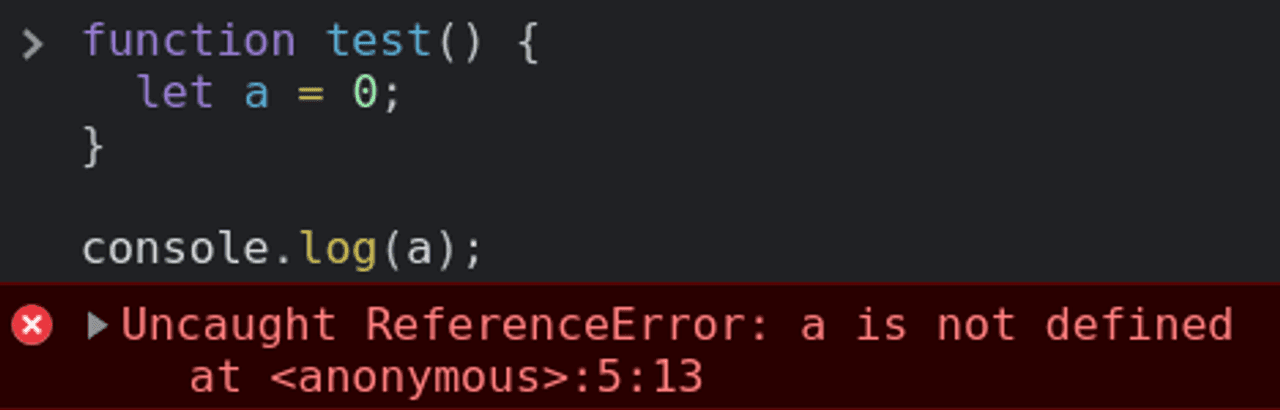This error occurs in several cases.

### Variable is not declared

``text.trim(); // Uncaught ReferenceError: text is not defined``

The variable `text` is not declared. To use the string method `trim()` the variable `text` variable must be declared and initialized with a string.

``let text = " Test ";text.trim(); // "Test"``

``function test() {  let a = 0;}console.log(a); // Uncaught ReferenceError: a is not defined``

The variable `a` must be made available for use where the error occurs.

``let a = 0;function test() {  a = 1;}console.log(a); // 0test();console.log(a); // 1``

## RangeError: Maximum call stack size exceeded

This error occurs when an infinite recursive function is called. Such a function has no exit from it or it does not apply.

``function countDown(fromNumber) {  console.log(fromNumber);  countDown(fromNumber - 1); // RangeError: Maximum call stack size exceeded}countDown(10);``

To fix this error, we need to add a recursion exit condition to the `countDown()` function.

``function countDown(fromNumber) {  console.log(fromNumber);  let nextNumber = fromNumber - 1;  if (nextNumber > 0) {    countDown(nextNumber);  }}countDown(10);``

## RangeError: precision is out of range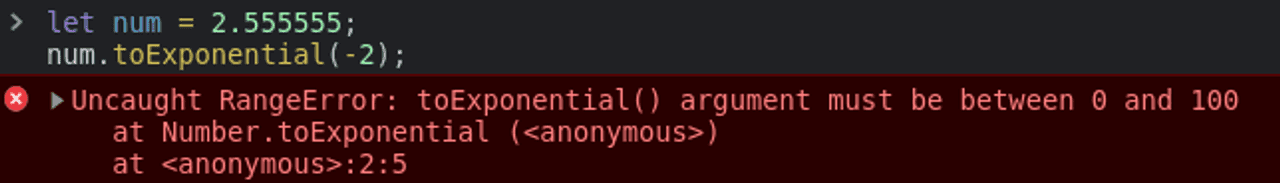An error occurs when a number outside the valid range is passed to `toExponential()`, `toFixed()`, or `toPrecision()`.

``let num = 4.777777;num.toExponential(-2); // RangeError: toExponential() argument must be between 0 and 100num = 4.8888;num.toFixed(105);  // RangeError: toFixed() digits argument must be between 0 and 100num = 4.1234;num.toPrecision(0);  // RangeError: toPrecision() argument must be between 1 and 100``

To correct, we will use the allowable values.

``let num = 4.777777;num.toExponential(4); // 2.5556e+0num = 4.8888;num.toFixed(2); // 3.00num = 4.1234;num.toPrecision(1); // 4``

## RangeError: invalid array length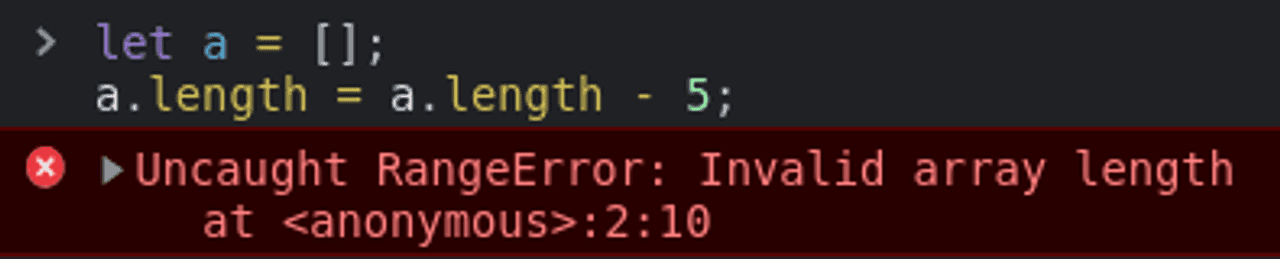This error occurs if we set the array to an invalid size: negative or greater than 232.

``new Array(-10); // RangeError: Invalid array lengthlet a = [];a.length = a.length - 5; // RangeError: Invalid array length``

To solve this error, we need to use a valid array length.

``new Array(10); // [empty × 10]``

## Summary

I hope you have learned something new for yourself. And you will be able to identify the cause of errors in JavaScript and how to fix them. These errors can occur in working projects and it is better to have an understanding of them beforehand.